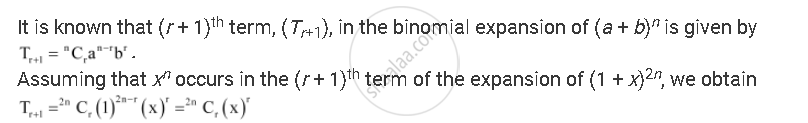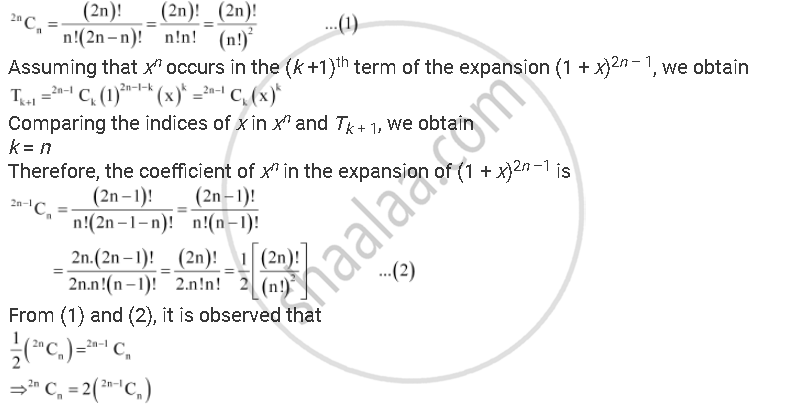CBSE (Arts) Class 11CBSE
Share
Notifications

View all notifications
Books Shortlist
Your shortlist is empty

# Prove that the Coefficient of Xn in the Expansion of (1 + X)2n is Twice the Coefficient of Xn in the Expansion of (1 + X)2n–1 . - CBSE (Arts) Class 11 - Mathematics

Login
Create free account

Forgot password?
ConceptGeneral and Middle Terms

#### Question

Prove that the coefficient of xn in the expansion of (1 + x)2n is twice the coefficient of xn in the expansion of (1 + x)2n–1 .

#### SolutionComparing the indices of x in xn and in Tr + 1, we obtain

r = n

Therefore, the coefficient of xn in the expansion of (1 + x)2n isTherefore, the coefficient of xn in the expansion of (1 + x)2n is twice the coefficient of xn in the expansion of (1 + x)2n–1.

Hence, proved.

Is there an error in this question or solution?

#### APPEARS IN

Solution Prove that the Coefficient of Xn in the Expansion of (1 + X)2n is Twice the Coefficient of Xn in the Expansion of (1 + X)2n–1 . Concept: General and Middle Terms.
S